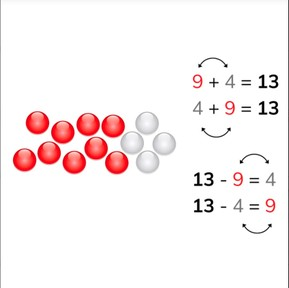Addition and subtraction to 20 using fact families

# Addition and subtraction to 20 using fact families

No account needed.8,000 schools use Gynzy92,000 teachers use Gynzy1,600,000 students use Gynzy

## General

Students learn to add and subtract numbers to 20 using their knowledge of fact families. They learn that each addition problem has a matching inverse subtraction problem.

1.OA.B.3
1.OA.B.4

## Relevance

These strategies help students to calculate quickly and easily. This helps strengthen the insight and understanding of math problems with numbers to 20.

## Introduction

Start by decomposing the numbers given into their respective amounts of beads. Then decompose the numbers showing their number bonds. Students can decompose the number with you with blocks at their tables. You can also ask students to decompose a few numbers out loud in which you ask them to decompose a given number. Example: I decompose the number 5 into 4 and .... Then determine which math problem is shown on the board. You see 15 bowls. 9 have been dried and 6 are still wet. A correct math problem would be 9 bowls and 6 bowls added. Discuss why the other options are wrong (for example 9 - 6 is wrong, because the total amount of bowls is 15, not 9.).

## Development

Show students the image of 13 beads, of which 9 are red and 4 are white. Tell students that you can make addition and subtraction problems that belong together. The numbers stay the same, but their position in the math problem gets swapped. In this case, 9 + 4 = 13, 4 + 9 = 13, 13 - 4 = 9 and 13 - 9 = 4 belong together. Practice this with other numbers. Use the number bonds to show how 5 can be decomposed. Using these numbers you can make four math problems, two addition and two subtraction problems. Tell students that they can think about the beads, 3 red and 8 white. Practice this with other numbers. Then challenge students to try to do this without beads or number bonds visible on the board.

Check that students are able to use fact families with numbers to 20 for addition and subtraction problems by asking the following questions:
- 13 + 4 = 17. What is the second addition problem that is a partner to this one?
- 19 - 3 = 16, which means that 19 - 16 = ...?
- 5 + 8 = 13, which means that 13 - 5 = ...?

## Guided practice

Students are first given problems in which they can count the objects as support. They are then given number bonds to use in solving the addition and subtraction problems.

## Closing

.Discuss the learning goal with students, and emphasize that by being able to use the fact families, that they make math problems easier and faster to solve. To close the lesson, solve two problems that belong to the given image as a class. Then repeat, but with a problem in which you can't see the number division.

## Teaching tips

Students who have difficulty with this learning goal can make use of MAB blocks, or manipulatives or a rekenrek. Ask them to decompose the number 14 into 9 and 5 blocks. Have them set the blocks down and tell which addition problems they can make with these blocks (9 + 5 = 14 and 5 + 9 = 14). Then ask what is left if you take 5 blocks away from 14 (14 - 5 = ?) and what is left if they take 9 blocks away from 14 (14 - 9 = ?). Remind them that they don't need to recalculate because they already know how many blocks there are as a result of the addition problem.

## Instruction materials

Optional: MAB blocks, manipulatives, rekenrek

### The online teaching platform for interactive whiteboards and displays in schools

• Save time building lessons

• Manage the classroom more efficiently

• Increase student engagement